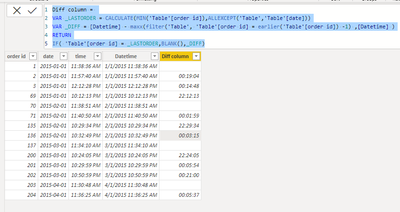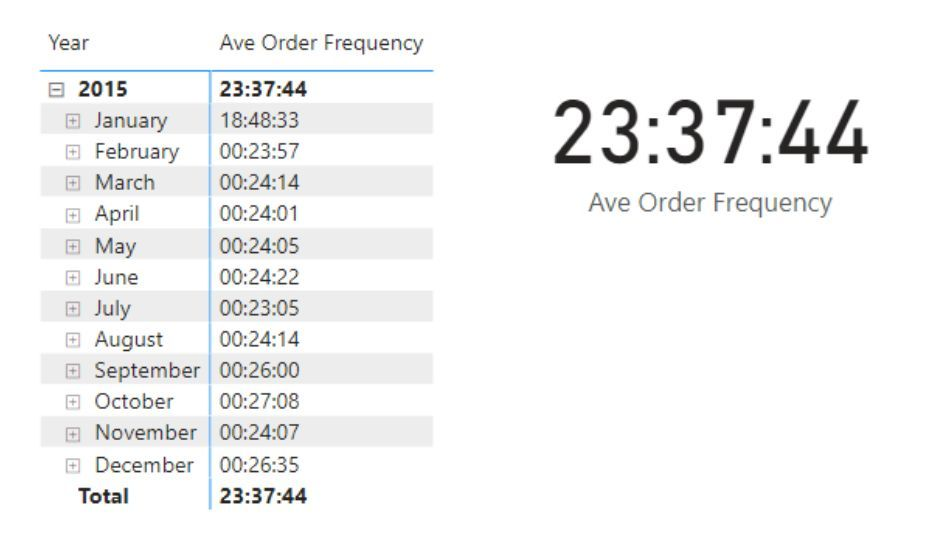cancel
Showing results for
Did you mean:Frequent Visitor

## Calculate Average Order frequency

Hi All,

I have dataset like below, I want to show =Average order frequency.

Like, we are reciving the order for every X minutes.

I have tried different calculation as suggested by in the forum. But, couldn't get the expected result.2 ACCEPTED SOLUTIONSCommunity Support

Here I suggest you to try this code to create a calculated column.

``````Diff column =
VAR _LASTORDER =
CALCULATE ( MIN ( 'Table'[order id] ), ALLEXCEPT ( 'Table', 'Table'[date] ) )
VAR _DIFF =
[Datetime]
- MAXX (
FILTER ( 'Table', 'Table'[order id] = EARLIER ( 'Table'[order id] ) - 1 ),
[Datetime]
)
RETURN
IF ( 'Table'[order id] = _LASTORDER, BLANK (), _DIFF )``````

Result is as below.Best Regards,
Rico Zhou

If this post helps, then please consider Accept it as the solution to help the other members find it more quickly.Solution Sage

it is a best practice to avoid using EARLIER to make the code easier to author and maintain, using variable (VAR), like in this example:

Diff column =
VAR FirstOrder_of_the_Day =
CALCULATE (
MIN ( 'Table'[Order ID] ),
ALLEXCEPT ( 'Table', 'Table'[Date] )
)

VAR Order_ID = 'Table'[Order ID]

VAR Diff =
[DateTime]
- MAXX (
FILTER ('Table',
'Table'[Order ID] = Order_ID - 1
),
[DateTime]
)
RETURN
IF (
'Table'[Order ID] = FirstOrder_of_the_Day,
BLANK(),
Diff
)

5 REPLIES 5Solution Sage

it is a best practice to avoid using EARLIER to make the code easier to author and maintain, using variable (VAR), like in this example:

Diff column =
VAR FirstOrder_of_the_Day =
CALCULATE (
MIN ( 'Table'[Order ID] ),
ALLEXCEPT ( 'Table', 'Table'[Date] )
)

VAR Order_ID = 'Table'[Order ID]

VAR Diff =
[DateTime]
- MAXX (
FILTER ('Table',
'Table'[Order ID] = Order_ID - 1
),
[DateTime]
)
RETURN
IF (
'Table'[Order ID] = FirstOrder_of_the_Day,
BLANK(),
Diff
)Super User

@Adhavan , In case Order ID are incremental only

a new column

Datetime= [Date]+ [Time]

Diff column =

[Datetime] - maxx(filter(Table, [Order_id] = earlier([Order_id]) -1) ,[Datetime] )

a Measure

Average(Table[Diff column])Frequent Visitor

Its provided the result at each column. But, it calculate wrong average value due to following reason, which I couldn't elimate nor find the solution.

1. It calculates 18 hours for Jan' 1st alone.

2. It showing 12+ hours for every first order of the day (calculating from previous day)

I have attached the reference image. Could you help me to rid out of that.Community Support

Here I suggest you to try this code to create a calculated column.

``````Diff column =
VAR _LASTORDER =
CALCULATE ( MIN ( 'Table'[order id] ), ALLEXCEPT ( 'Table', 'Table'[date] ) )
VAR _DIFF =
[Datetime]
- MAXX (
FILTER ( 'Table', 'Table'[order id] = EARLIER ( 'Table'[order id] ) - 1 ),
[Datetime]
)
RETURN
IF ( 'Table'[order id] = _LASTORDER, BLANK (), _DIFF )``````

Result is as below.Best Regards,
Rico Zhou

If this post helps, then please consider Accept it as the solution to help the other members find it more quickly.Frequent Visitor

Thanks a lot.

It shows the exact result I want.

Thank,

Mani